This book is archived and will be removed July 6, 2022. Please use the updated version.

Gauss’s Law

# 38 Explaining Gauss’s Law

### Learning Objectives

By the end of this section, you will be able to:

• State Gauss’s law
• Explain the conditions under which Gauss’s law may be used
• Apply Gauss’s law in appropriate systems

We can now determine the electric flux through an arbitrary closed surface due to an arbitrary charge distribution. We found that if a closed surface does not have any charge inside where an electric field line can terminate, then any electric field line entering the surface at one point must necessarily exit at some other point of the surface. Therefore, if a closed surface does not have any charges inside the enclosed volume, then the electric flux through the surface is zero. Now, what happens to the electric flux if there are some charges inside the enclosed volume? Gauss’s law gives a quantitative answer to this question.

To get a feel for what to expect, let’s calculate the electric flux through a spherical surface around a positive point charge q, since we already know the electric field in such a situation. Recall that when we place the point charge at the origin of a coordinate system, the electric field at a point P that is at a distance r from the charge at the origin is given by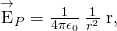whereis the radial vector from the charge at the origin to the point P. We can use this electric field to find the flux through the spherical surface of radius r, as shown in (Figure).

A closed spherical surface surrounding a point charge q.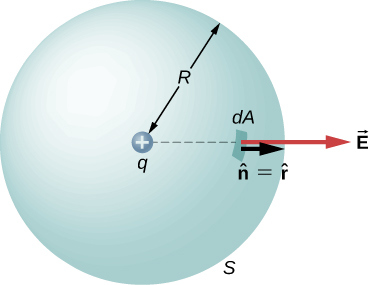Then we apply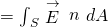to this system and substitute known values. On the sphere,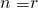and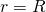, so for an infinitesimal area dA,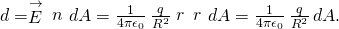We now find the net flux by integrating this flux over the surface of the sphere: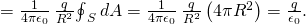where the total surface area of the spherical surface is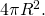This gives the flux through the closed spherical surface at radius r as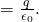A remarkable fact about this equation is that the flux is independent of the size of the spherical surface. This can be directly attributed to the fact that the electric field of a point charge decreases as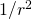with distance, which just cancels the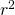rate of increase of the surface area.

### Electric Field Lines Picture

An alternative way to see why the flux through a closed spherical surface is independent of the radius of the surface is to look at the electric field lines. Note that every field line from q that pierces the surface at radius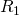also pierces the surface at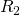((Figure)).

Flux through spherical surfaces of radiiandenclosing a charge q are equal, independent of the size of the surface, since all E-field lines that pierce one surface from the inside to outside direction also pierce the other surface in the same direction.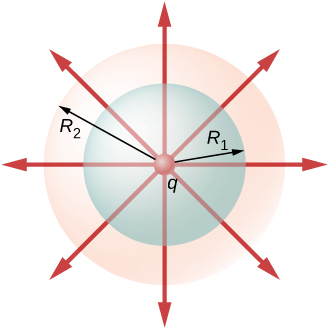Therefore, the net number of electric field lines passing through the two surfaces from the inside to outside direction is equal. This net number of electric field lines, which is obtained by subtracting the number of lines in the direction from outside to inside from the number of lines in the direction from inside to outside gives a visual measure of the electric flux through the surfaces.

You can see that if no charges are included within a closed surface, then the electric flux through it must be zero. A typical field line enters the surface at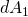and leaves at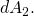Every line that enters the surface must also leave that surface. Hence the net “flow” of the field lines into or out of the surface is zero ((Figure)(a)). The same thing happens if charges of equal and opposite sign are included inside the closed surface, so that the total charge included is zero (part (b)). A surface that includes the same amount of charge has the same number of field lines crossing it, regardless of the shape or size of the surface, as long as the surface encloses the same amount of charge (part (c)).

Understanding the flux in terms of field lines. (a) The electric flux through a closed surface due to a charge outside that surface is zero. (b) Charges are enclosed, but because the net charge included is zero, the net flux through the closed surface is also zero. (c) The shape and size of the surfaces that enclose a charge does not matter because all surfaces enclosing the same charge have the same flux.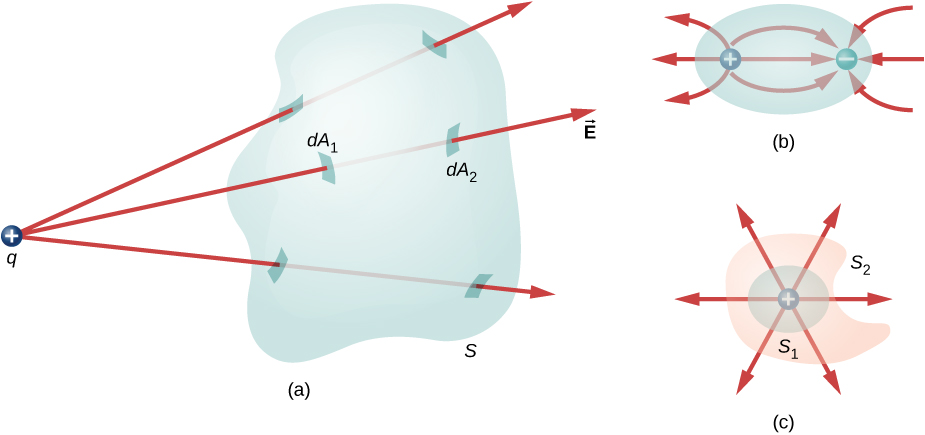### Statement of Gauss’s Law

Gauss’s law generalizes this result to the case of any number of charges and any location of the charges in the space inside the closed surface. According to Gauss’s law, the flux of the electric field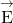through any closed surface, also called a Gaussian surface, is equal to the net charge enclosed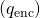divided by the permittivity of free space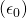: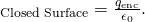This equation holds for charges of either sign, because we define the area vector of a closed surface to point outward. If the enclosed charge is negative (see (Figure)(b)), then the flux through either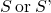is negative.

The electric flux through any closed surface surrounding a point charge q is given by Gauss’s law. (a) Enclosed charge is positive. (b) Enclosed charge is negative.The Gaussian surface does not need to correspond to a real, physical object; indeed, it rarely will. It is a mathematical construct that may be of any shape, provided that it is closed. However, since our goal is to integrate the flux over it, we tend to choose shapes that are highly symmetrical.

If the charges are discrete point charges, then we just add them. If the charge is described by a continuous distribution, then we need to integrate appropriately to find the total charge that resides inside the enclosed volume. For example, the flux through the Gaussian surface S of (Figure) is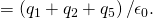Note that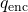is simply the sum of the point charges. If the charge distribution were continuous, we would need to integrate appropriately to compute the total charge within the Gaussian surface.

The flux through the Gaussian surface shown, due to the charge distribution, is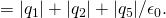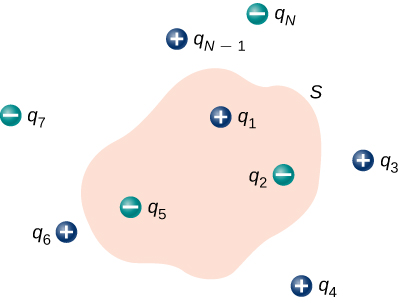Recall that the principle of superposition holds for the electric field. Therefore, the total electric field at any point, including those on the chosen Gaussian surface, is the sum of all the electric fields present at this point. This allows us to write Gauss’s law in terms of the total electric field.

Gauss’s Law

The fluxof the electric fieldthrough any closed surface S (a Gaussian surface) is equal to the net charge encloseddivided by the permittivity of free space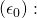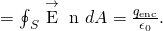To use Gauss’s law effectively, you must have a clear understanding of what each term in the equation represents. The fieldis the total electric field at every point on the Gaussian surface. This total field includes contributions from charges both inside and outside the Gaussian surface. However,is just the charge inside the Gaussian surface. Finally, the Gaussian surface is any closed surface in space. That surface can coincide with the actual surface of a conductor, or it can be an imaginary geometric surface. The only requirement imposed on a Gaussian surface is that it be closed ((Figure)).

A Klein bottle partially filled with a liquid. Could the Klein bottle be used as a Gaussian surface?Electric Flux through Gaussian Surfaces Calculate the electric flux through each Gaussian surface shown in (Figure).

Various Gaussian surfaces and charges.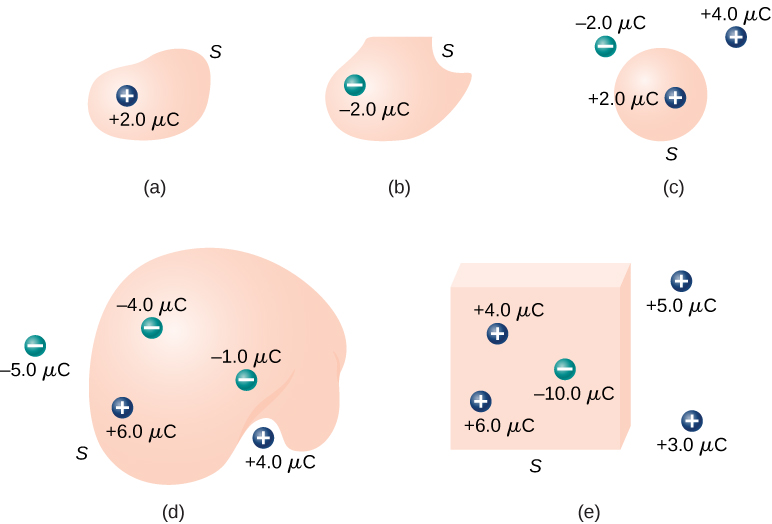Strategy From Gauss’s law, the flux through each surface is given by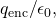whereis the charge enclosed by that surface.

Solution For the surfaces and charges shown, we find

1.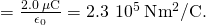2.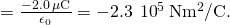3.4.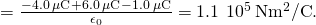5.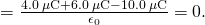Significance In the special case of a closed surface, the flux calculations become a sum of charges. In the next section, this will allow us to work with more complex systems.

Check Your Understanding Calculate the electric flux through the closed cubical surface for each charge distribution shown in (Figure).

A cubical Gaussian surface with various charge distributions.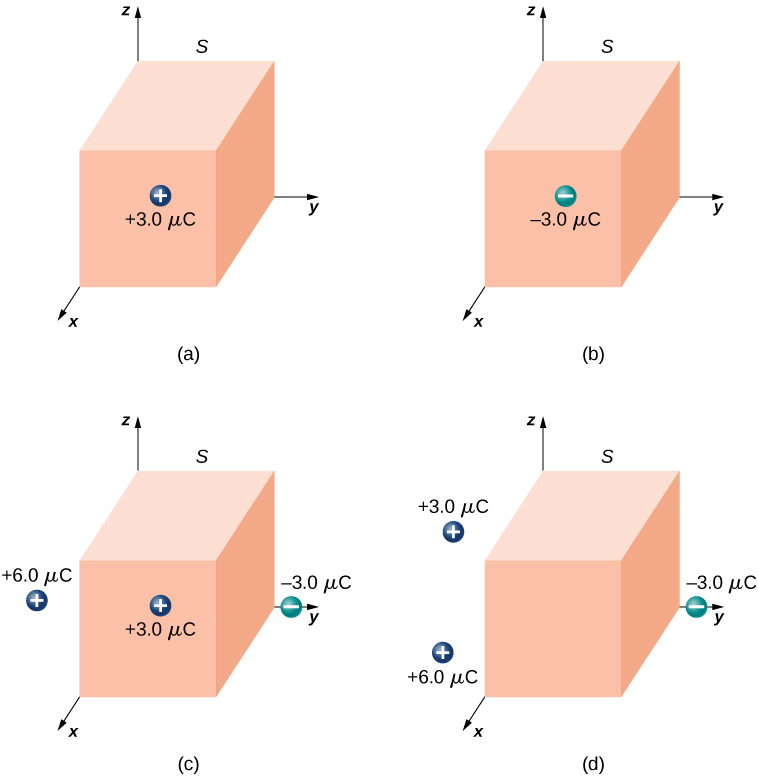a.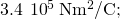b.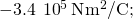c.d. 0

Use this simulation to adjust the magnitude of the charge and the radius of the Gaussian surface around it. See how this affects the total flux and the magnitude of the electric field at the Gaussian surface.

### Summary

• Gauss’s law relates the electric flux through a closed surface to the net charge within that surface,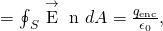whereis the total charge inside the Gaussian surface S.

• All surfaces that include the same amount of charge have the same number of field lines crossing it, regardless of the shape or size of the surface, as long as the surfaces enclose the same amount of charge.

### Conceptual Questions

Two concentric spherical surfaces enclose a point charge q. The radius of the outer sphere is twice that of the inner one. Compare the electric fluxes crossing the two surfaces.

Since the electric field vector has a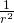dependence, the fluxes are the same since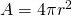.

Compare the electric flux through the surface of a cube of side length a that has a charge q at its center to the flux through a spherical surface of radius a with a charge q at its center.

(a) If the electric flux through a closed surface is zero, is the electric field necessarily zero at all points on the surface? (b) What is the net charge inside the surface?

a. no; b. zero

Discuss how Gauss’s law would be affected if the electric field of a point charge did not vary as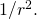Discuss the similarities and differences between the gravitational field of a point mass m and the electric field of a point charge q.

Both fields vary as. Because the gravitational constant is so much smaller than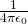, the gravitational field is orders of magnitude weaker than the electric field. Also, the gravitational flux through a closed surface is zero or positive; however, the electric flux is positive, negative, or zero, depending on the definition of flux for the given situation.

Discuss whether Gauss’s law can be applied to other forces, and if so, which ones.

Is the term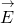in Gauss’s law the electric field produced by just the charge inside the Gaussian surface?

No, it is produced by all charges both inside and outside the Gaussian surface.

Reformulate Gauss’s law by choosing the unit normal of the Gaussian surface to be the one directed inward.

### Problems

Determine the electric flux through each surface whose cross-section is shown below.Find the electric flux through the closed surface whose cross-sections are shown below.a.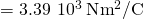; b.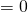;
c.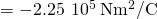;
d.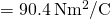A point charge q is located at the center of a cube whose sides are of length a. If there are no other charges in this system, what is the electric flux through one face of the cube?

A point charge of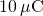is at an unspecified location inside a cube of side 2 cm. Find the net electric flux though the surfaces of the cube.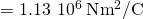A net flux of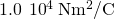passes inward through the surface of a sphere of radius 5 cm. (a) How much charge is inside the sphere? (b) How precisely can we determine the location of the charge from this information?

A charge q is placed at one of the corners of a cube of side a, as shown below. Find the magnitude of the electric flux through the shaded face due to q. Assume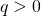.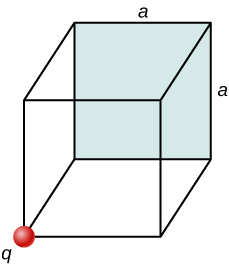Make a cube with q at the center, using the cube of side a. This would take four cubes of side a to make one side of the large cube. The shaded side of the small cube would be 1/24th of the total area of the large cube; therefore, the flux through the shaded area would be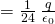.

The electric flux through a cubical box 8.0 cm on a side is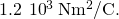What is the total charge enclosed by the box?

The electric flux through a spherical surface is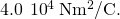What is the net charge enclosed by the surface?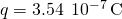A cube whose sides are of length d is placed in a uniform electric field of magnitude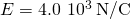so that the field is perpendicular to two opposite faces of the cube. What is the net flux through the cube?

Repeat the previous problem, assuming that the electric field is directed along a body diagonal of the cube.

zero, also because flux in equals flux out

A total charge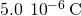is distributed uniformly throughout a cubical volume whose edges are 8.0 cm long. (a) What is the charge density in the cube? (b) What is the electric flux through a cube with 12.0-cm edges that is concentric with the charge distribution? (c) Do the same calculation for cubes whose edges are 10.0 cm long and 5.0 cm long. (d) What is the electric flux through a spherical surface of radius 3.0 cm that is also concentric with the charge distribution?

### Glossary

Gaussian surface
any enclosed (usually imaginary) surface